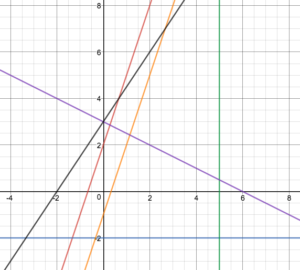## What you’ll learn to do: Evaluate linear equations

Now that we have learned how to plot points on a coordinate plane and graph linear equations, we can begin to analyze the equations of lines and evaluate the different characteristics of these lines. In this section, we will learn about the commonly used forms for writing linear equations and the properties of lines that can be determined from their equations.

For example, without creating a table of values, you will be able to match each equation below to its corresponding graph. You will also be able to explain the similarities and differences of each line, how they relate to each other, and why they behave that way.(a) $y=3x+2$

(b) $y-4=-\frac{1}{2}(x+2)$

(c) $x=5$

(d) $y=-2$

(e) $3x=y+1$

(f) $2y-3x=6$

## Contribute!

Did you have an idea for improving this content? We’d love your input.